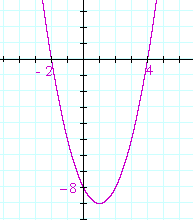# Section 3:   The graph of y = A quadratic

Back to Section 2

Back to Section 1

THE GRAPH OF EVERY QUADRATIC is the figure known as a parabola.As the graph of any equation, every coördinate pair (xy) solves the equation.  (Compare Lesson 33.)

Problem 1.

a)  Is (2, −10) on the graph of  y = x² − x − 12?

Do the problem yourself first!

Yes. Because when x = 2, then y = 4 − 2 − 12 = −10. Those coördinates solve the quadratic equation.

b)  Is (2, 10) on that graph?

No. We already found that when x = 2,  y = −10. Corresponding to each value of x, there is one and only one value of y.

Problem 2.   What is the relationship between the roots of a quadratic and the x-intercepts of its graph?

The roots are the x-intercepts!  They are the values of x that make the quadratic equal to 0.   y = 0.  They are the solutions to the quadratic equation.

The y-intercept of the graph is the constant term, c.  It is the value of y when x  =  0.

Example 1.   Here is the graph of  y = x² − 2x − 15.x² − 2x − 15  =  (x + 3)(x − 5)

The roots -- the x-intercepts -- are −3 and 5.

The y-intercept is the constant term, −15.

For the graph of a parabola that begins  −x², see Topic 15 of Precalculus:  Reflections about the x-axis.

Example 2.  A quadratic inequality.   For which values of x is

x² − 2x − 15 < 0 ?

Solution.  This is the quadratic of Example 1.  Look at its graph.  Where is it negative, that is, below the x-axis?

It's negative between the roots.  That is, that quadratic is negative -- less than 0 -- when x is between −3 and 5:  when −3 < x < 5.

(For the meaning of that continued inequality, see Lesson 11.)

Similarly, we could ask for which values of x is

x² − 2x − 15 > 0 ?

Look at the graph.  That quadratic is positive -- the graph is above the x-axis -- when  x < −3  or  x > 5.

We have now covered the three possibilities:

That quadratic is equal to 0 at the two roots.

It is less than 0 between the two roots.

It is greater than 0 to the left and right of the two roots.

These three possiblities, which are true for any real number, has the fancy name of the Law of Trichotomy.  Any number must be either equal to, less than, or greater than 0.

The Law of Trichotomy also takes this form:

For any real numbers a, b, either a = b, a < b, or a > b.

We must be able to know, though, which of those possibilities is true. For any two numbers, we must be able to know their relative order. That is inherent in the meaning of a "number."

Example 3.

How many real roots, i.e. roots that are real numbers, has the quadratic of each graph?Answer.   Graph a) has two real roots.  It has two x-intercepts.

Graph b) has no real roots.  It has no x-intercepts.  Both roots are complex.

Graph c) has two real roots.  But they are a double root.  The graph touches but does not cross the x-axis.

In which graph is the discriminant positive?   Graph a).

In which graph is the discriminant negative?   Graph b).

In which graph is the discriminant 0?   Graph c).

Problem 3.   Sketch the graph of  y = x² − 2x − 8.  That is, show the x- and y-intercepts.x² −2x − 8 = (x + 2)(x − 4).
The roots are −2, 4.  The y-intercept is the constant term, − 8.

Problem 4.

x² − 2x − 8 > 0.

This is the quadratic of Problem 3.  The graph is positive when x < − 2  or  x > 4.

b)  Solve  x² − 2x − 8 < 0.

The graph is negative when −2 < x < 4.

Problem 5.   Sketch the graph of  y = x² + 4x + 4.x² + 4x + 4 = (x + 2)².  There is a double root at −2.  The y-intercept is the constant term, 4.

Problem 6.   Sketch the graph of  y = x² + 3x.x² + 3x = x(x + 3). Therefore, the roots are 0, −3.
Since the constant term is 0, this graph passes through the origin.

Problem 7.   Sketch the graph of  y = 3x² − 5x − 2.3x² − 5x − 2 = (3x + 1)(x − 2). The roots are −1/3,  2. The constant term is −2.

Back to Section 1

Back to Section 2Next Lesson:  Logarithms

Please make a donation to keep TheMathPage online.
Even \$1 will help.